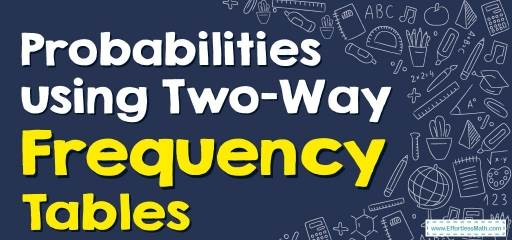# How to Find Probabilities using Two-Way Frequency Tables?In this article, you will learn how to find probabilities using Two-Way Frequency Tables in a few simple steps.

## Step by step guide to Find Probabilities using Two-Way Frequency Tables

The information obtained from research is often a mass of raw, meaningless, and disordered information that makes it impossible to draw any conclusions or interpret it. Therefore, for any type of data analysis, it is necessary to classify the data according to a logical order to make it meaningful and interpretable. Classification of data requires the step-by-step calculation of the amplitude of changes, number of classes, class interval, types of frequencies using specific formulas. In data classification, all the information is collected in a table called Frequency Table, and this table should be the basis for calculating the Central Index, Dispersion Index and comparing a group of data with other groups for statistical inference.

The frequency table consists of One category for rows and the other for columns. Each row represents the characteristics of a class or category. The attributes for each row are also listed in the columns. For example, if we consider blood type, ‌ The category name for each row is one of the blood groups such as $$A, AB, B$$ or $$O$$.

The number of iterations of any value in a category column is called the “frequency”. This value is placed in the frequency column.

If we divide the frequency of each category by the sum of the frequencies, we get the “relative frequency”. If we show the total number of frequencies in the frequency table by $$n$$, we can use the following mathematical relation to illustrate how the relative frequency is calculated:

$$ri=\frac{fi}{n}$$

### Finding Probabilities using Two-Way Frequency Tables Example 1:

Each student in a random sample of seniors at a local high school participated in a survey. These students were asked to indicate their gender and their eye color. The following table summarizes the results of the survey.

a) Suppose that one of these seniors is randomly selected. What is the probability that the selected student has green eyes?

b) Suppose that one of these seniors is randomly selected. What is the probability that the selected student is a female?

Solution:

a) $$\frac{number \ of students \ with \ green \ eyes}{number \ of \ students}=\frac{15}{139}$$

b) $$\frac{number \ of \ females}{number \ of \ students}=\frac{63}{139}$$

### Finding Probabilities using Two-Way Frequency Tables Example 2:

Students were asked what their hair color was, the results are shown below. If a boy is selected at random, find the probability that the boy has brown hair.

Solution: $$\frac{number \ of \ boys \ with \ brown \ hair}{number \ of \ students}=\frac{13}{99}$$

## Exercises for Finding Probabilities using Two-Way Frequency Tables

### Solve all the problems.

1) The two-way table shows the distribution of members of the audience at a play.

a) Complete the two-way tables.

b) What is the probability that a randomly chosen audience member is a child and is seated on the circle?

c) What is the probability that a randomly chosen audience member is a child?

2) The following table represents the data collected from 120 conference attendees of different nationalities:

a) Complete the two-way tables.

b) Find the probability that a randomly selected participant is an English-speaking woman.

1)

b) Probability: $$\frac{32}{115}$$

c) Probability: $$\frac{64}{115}$$

2)

b) Probability: $$\frac{17}{120}$$

### What people say about "How to Find Probabilities using Two-Way Frequency Tables? - Effortless Math: We Help Students Learn to LOVE Mathematics"?

No one replied yet.

X
30% OFF

Limited time only!

Save Over 30%

SAVE $5 It was$16.99 now it is \$11.99